﻿ 一次西南涡东移诱发的川渝暴雨诊断分析 Diagnostic Analysis of the Heavy Precipitation in Sichuan and Chongqing Caused by Eastward Shift of Southwest Vortex

Open Journal of Nature Science
Vol. 07  No. 03 ( 2019 ), Article ID: 30469 , 9 pages
10.12677/OJNS.2019.73035

Diagnostic Analysis of the Heavy Precipitation in Sichuan and Chongqing Caused by Eastward Shift of Southwest Vortex

Ziyue Wang

School of Atmospheric Sciences, Chengdu University of Information Technology, Chengdu SichuanReceived: May 7th, 2019; accepted: May 21st, 2019; published: May 28th, 2019ABSTRACT

By using the NCEP-FNL 1˚ × 1˚ reanalysis data and China automated station and CMORPH kour precipitation fusion products precipitation data, the circulation background, atmospheric dynamics, water vapor, thermal effects, and the non-geostrophic wet-Q vector were analyzed. The results show that the structure of the southwest vortex changes with its movement, and the rain belt is mainly distributed in the center of the low vortex and the right front of the low vortex. Precipitation intensity increases with southwest vortex intensity. Low-level convergence, high-level divergence and strong upward movement are found above precipitation center which provide the dynamic conditions for the development and maintenance of heavy rain. The lower tropospheric temperature in the lower troposphere decreases with height, indicating that a large amount of unstable energy is stored in the event of heavy rain. Warm and humid air from the Bay of Bengal and South China Sea provided sufficient water vapor for the storm. The convergence of wet-Q vector divergence coincides with the precipitation area during this precipitation, and the vortex center intensity and precipitation are positively correlated with their convergence strength, so they can be used to diagnose the torrential rain area and intensity.

Keywords:Southwest Vortex, Rainstorm, Wet-Q VectorCopyright © 2019 by author(s) and Hans Publishers Inc.1. 引言

2017年7月5~6日，受西南涡的影响，四川东部、重庆北部、陕西南部、山西南部、河南中西部以及山东西南部等地区出现了一次自西向东的区域性强降水过程，该降水过程时间较、强度大、影响范围广。5日，暴雨集中在四川东部；6日，西南低涡东移并有所加强，暴雨主要集中在重庆北部、陕西南部、河南中西部、山东西南部等地。本文利用中国自动站与CMORPH小时降水融合产品降水资料和NCEP FNL 1˚ × 1˚再分析资料，以及热力物理量、动力物理量、和非地转湿Q矢量等诊断方法对本次降水事件进行了一定的诊断分析。

2. 资料和方法

2.1. 资料

2.2. 方法

$Q=\left(Q{}_{x},{Q}_{y}\right)=\left(-\frac{\partial {V}_{g}}{\partial x}·\nabla \left(-\frac{\partial \phi }{\partial p}\right),-\frac{\partial {V}_{g}}{\partial y}·\nabla \left(-\frac{\partial \phi }{\partial p}\right)\right)$ (1)

Q矢量表示了作用在位温梯度上的地转变形的常数倍，在对流层下层，可近似地表示非地转运动。Q矢量不但可以诊断大气垂直环流，而且因其可代表流场和温度场热成风的个别变化，即决定了水平温度的个别变化，故可用于预报锋生或锋消，对预报天气提供了更加精确的分析方法。

Q*矢量采用张兴旺  等提出的计算方法，即在P坐标系考虑了水汽凝结潜热释放的非绝热作用后，其Q*矢量表达式为：

${Q}_{x}^{*}=\frac{1}{2}\left[f\left(\frac{\partial v}{\partial p}\frac{\partial u}{\partial x}-\frac{\partial u}{\partial p}\frac{\partial v}{\partial x}\right)-h\frac{\partial \stackrel{\to }{v}}{\partial x}·\nabla \theta +\frac{\partial \left(hH\right)}{\partial x}\right]$ (2)

${Q}_{y}^{*}=\frac{1}{2}\left[f\left(\frac{\partial v}{\partial p}\frac{\partial u}{\partial y}-\frac{\partial u}{\partial p}\frac{\partial v}{\partial y}\right)-h\frac{\partial \stackrel{\to }{V}}{\partial y}·\nabla \theta +\frac{\partial \left(hH\right)}{\partial y}\right]$ (3)

$\stackrel{\to }{{Q}^{*}}=\left({Q}_{x}^{*},{Q}_{y}^{*}\right)$ (4)

${f}^{2}\frac{{\partial }^{2}\omega }{\partial {p}^{2}}+{\nabla }^{2}\left(\sigma \omega \right)=-2\nabla ·{Q}^{*}$ (5)

$\frac{\partial {Q}_{x}^{*}}{\partial x}+\frac{\partial {Q}_{y}^{*}}{\partial y}=\nabla ·{Q}^{*}$ (6)

Q*矢量涡度表达式为：

${\xi }_{{Q}^{*}}=\frac{\partial {Q}_{y}^{*}}{\partial x}-\frac{\partial {Q}_{x}^{*}}{\partial y}$ (7)

3. 环流背景与天气过程概况

3.1. 环流背景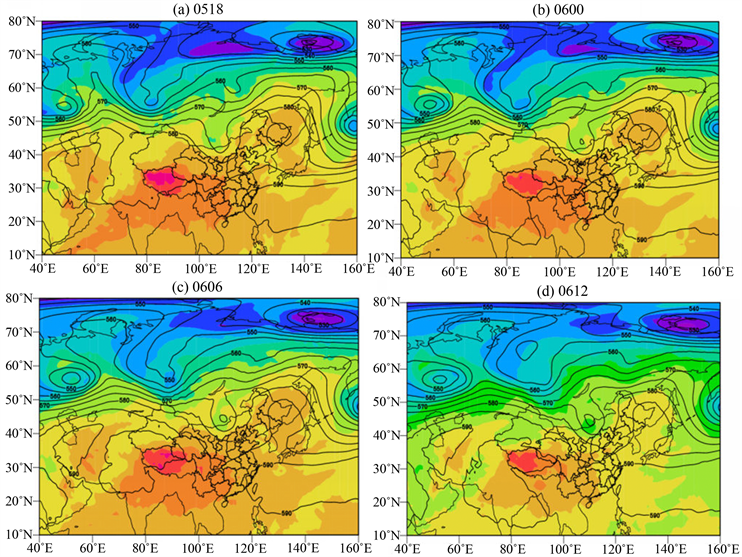Figure 1. Geopotential height and temperature at 500 hPa during 5-6 July, 2017

3.2. 降水实况分析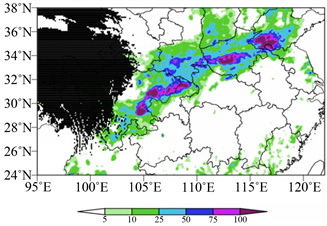Figure 2. 24 hours accumulated precipitation during 5-6 July, 2017

3.3. 西南低涡移动过程分析Figure 3. Precipitation and 700 hPa wind filed for per 6 hours during 5-6 July, 2017

4. 诊断分析

4.1. 涡度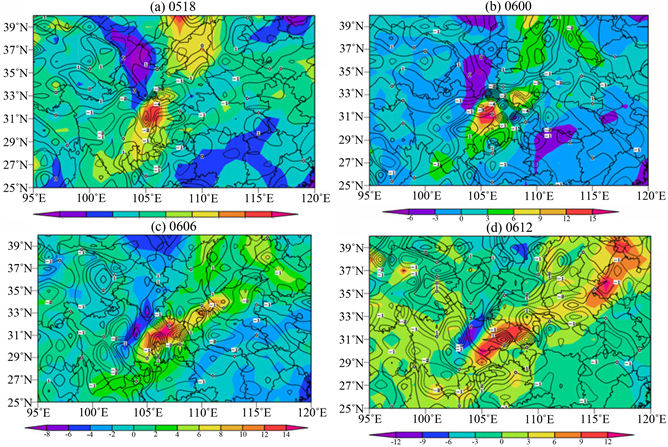Figure 4. The vorticity distribution during 5-6 July, 2017

4.2. 垂直速度

5日18时西南涡初生期降水较弱时(图5(a))，可以看到106˚~108˚E的800~350 hPa高度上存在较弱的上升运动。到6日00时，西南涡稳定维持，在106˚~107˚E的850~550 hPa高度范围内存在极强的上升运动，此时已产生较强降水。到6日06时(图5(c))，西南涡已达到鼎盛时期，垂直运动亦最强。6日12时(图5(c))西南涡开始减弱，此时的垂直运动也随之减弱。由此可见，垂直速度不断加强为暴雨提供有利的条件。

4.3. 假相当位温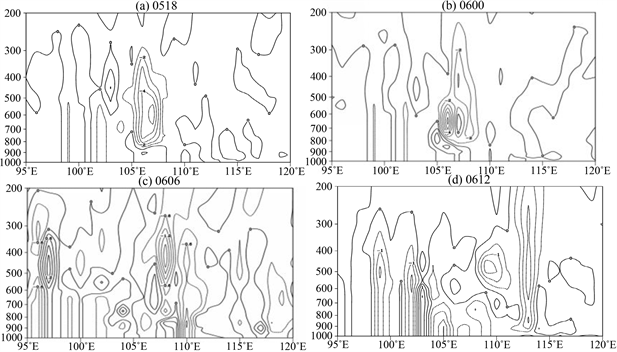Figure 5. The vertical velocity along 32˚N during 5-6 July, 2017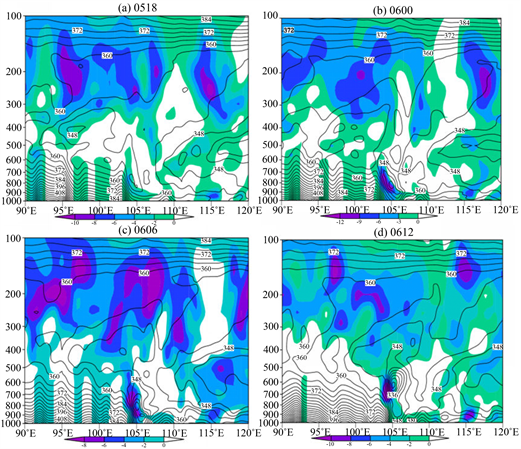Figure 6. The potential pseudo-equivalent temperature distribution during 5-6 July, 2017

4.4. 非地转湿Q矢量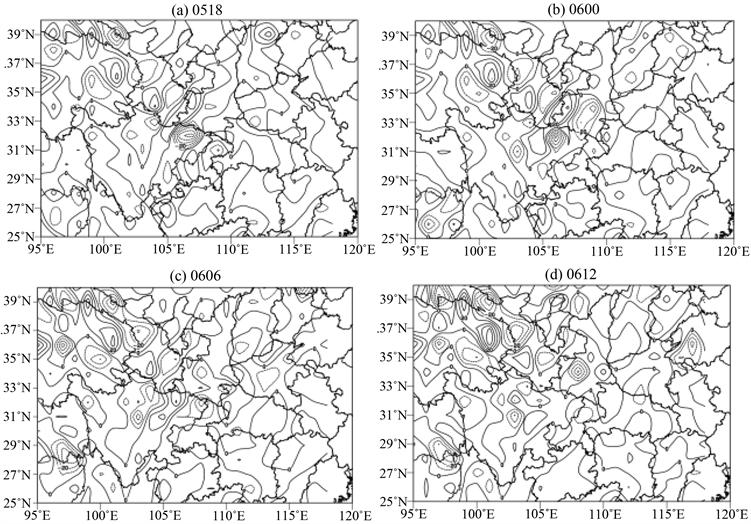Figure 7. The wet-Q vector distribution during 5-6 July, 2017

5. 结论

Diagnostic Analysis of the Heavy Precipitation in Sichuan and Chongqing Caused by Eastward Shift of Southwest Vortex[J]. 自然科学, 2019, 07(03): 254-262. https://doi.org/10.12677/OJNS.2019.73035

1. 1. 卢敬华. 西南低涡概论[M]. 北京: 气象出版社, 1986: 1-235.

2. 2. 朱乾根, 林锦瑞, 寿绍文, 唐东升. 天气学原理和方法[M]. 第四版. 北京: 气象出版社, 2015: 1-649.

3. 3. 高守亭. 流场配置及地形对西南低涡形成的动力作用[J]. 大气科学, 1987, 11(3): 39-47.

4. 4. 濮梅娟, 刘富明, 沈如金. 一次夏季西南低涡形成机理的数值试验[J]. 高原气象, 1989, 8(4): 321-330.

5. 5. 陈忠明, 徐茂良, 闵文彬. 1998年夏季西南低涡活动与长江上游暴雨[J]. 高原气象, 2003, 22(2): 162-167.

6. 6. 张政泰, 林明宇, 亓鹏, 陈圣哲, 王澄海. 西南涡发生发展机制中热力强迫作用的数值试验[J]. 干旱气象, 2016, 34(3): 533-539.

7. 7. 张小峰, 刘元珺, 徐娟娟, 何豫秦, 王云鹏, 彭菊蓉, 杨睿敏. 西南低涡背景下秦巴山区强降水特征[J]. 安徽农业科学, 2016, 539(34): 191-193.

8. 8. 岳彩军, 董美莹, 寿绍文, 姚秀萍. 改进的湿Q矢量分析方法及梅雨锋暴雨形成机制[J]．高原气象, 2007, 26(1): 165-175.

9. 9. 梁琳琳, 寿绍文, 苗春生. 应用湿Q矢量分解理论诊断分析“05. 7”梅雨锋暴雨[J]. 南京气象学院学报, 2008, 31(2): 167-175.

10. 10. 刘运成. 青藏高原东侧一次连续大暴雨过程湿Q矢量分析[J]. 气象, 2006, 32(12): 43-49.

11. 11. 段海霞, 陆维松, 毕宝贵. “049”川渝暴雨的湿Q矢量与湿位涡分析[J]. 南京气象学院学报, 2007, 30(5): 674-680.

12. 12. 李国平, 刘行军. 西南低涡暴雨的湿位涡诊断分析[J]. 应用气象学报, 1994, 5(3): 354-360.

13. 13. Hoskins, B.J., Draghici, I. and Davies, H.C. (1978) A New Look at the M-Equation. Quarterly Journal of the Royal Meteorological Society, 104, 31-38. https://doi.org/10.1002/qj.49710443903

14. 14. 张兴旺. 湿Q矢量表达式及其应用[J]. 气象, 1998, 24(8): 3-7.